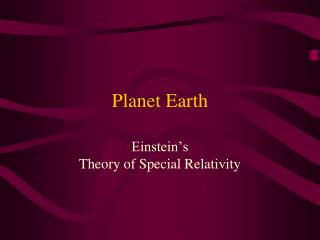DownloadDownload PresentationPlanet Earth

# Planet Earth

Télécharger la présentation## Planet Earth

- - - - - - - - - - - - - - - - - - - - - - - - - - - E N D - - - - - - - - - - - - - - - - - - - - - - - - - - -
##### Presentation Transcript

1. Planet Earth Einstein’s Theory of Special Relativity

2. Classical Physics Review • Length and Time • Define a standard for each and compare the unknowns to them. • Kinematics • derive from length and time velocity and acceleration • v =  x/ t • a = v/ t • If a is constant • v = at and x = 1/2 a t2 • as t goes to infinity v goes to infinity

3. Dynamics • Newton’s Laws (existence and strength of all forces • Law of inertia • F = ma • Law of mutual interaction • Conservation laws • Momentum • Energy • Electricity and Magnetism • Maxwell’s equations for the dynamics of charged particles

4. Implicit Assumption • All the laws of nature have the same form in all frames of reference moving at constant velocity with respect to each other. • Transformation Laws between frames. S0 S X0 vt x X0 = x – v t and V0 = V - v

5. Trouble • All observers can use the same laws, and communicate! • Michelson-Morley Experiment • to study the nature of light - particle or wave • if it is a wave - what is the nature of the medium? • search for the ether • the experiment was null

6. Einstein’s Postulates • All the laws of nature have the same form in all frames of reference moving at constant velocity with respect to each other. • The speed of light is a constant for all observers moving at constant velocity with respect to each other.

7. New Transformation Equations •  t =  t0 / (1 - v2/c2)1/2 •  x =  x0 (1 - v2/c2)1/2 • m = m0 / (1 - v2/c2)1/2 • V’x = (Vx - v) / (1 - Vx  v/c2)

8. Time dilation t =  t0 / (1 - v2/c2)1/2 • A space ship goes by at v = .8c. Someone on the ship drops a ball 16 ft. and measures a time of flight of 1 sec ( t0) . What does a stationary observer measure ( t)? •  t =  t0 / (1 - v2/c2)1/2 = 1/(1 - .64)1/2 = 1.67 sec. 16 ft.

9. Length Contraction x =  x0 (1 - v2/c2)1/2 • If the ball on the spaceship has a diameter of 5 cm ( x0 )as measured by someone on the ship. What is its diameter as measured by a stationary observer ( x)? •  x =  x0 (1 - v2/c2)1/2 = 5 (1 - .64)1/2 = 5  .6 = 3cm 16 ft.

10. Mass Increasem = m0 /(1 - v2/c2)1/2 • Same ball has a mass of 10 gm as measured by someone on the ship(m0). What is the mass as measured by the stationary observer (m)? • m = m0 /(1 - v2/c2)1/2 = 10/(1 - .64)1/2 = 10/ .6 = 16.7 gm 16 ft.

11. Addition of velocityV’x = (Vx - v) / (1 - Vx  v/c2) • A space ship goes by at .8c (v) and watches someone on earth turn on a flashlight. What is the speed of the light as measured by the observer on the ship? Note the result is independent of v! • V’x = (Vx - v) / (1 - Vx  v/c2) = ( c - .8c)/(1 - c .8c/c2) = c S S’ V = .8c

12. Compare fictitious space ship speed with actual escape speed • Fictitious speed of .8 c gives the results on the previous slides. • Actual escape speed is about 7 mi/sec. The speed of light is 186,000 mi/sec. • The escape speed can be written as .00004c • try to redo the previous slides with this speed instead of .8c! • For everyday experience v/c is very small and Einstein’s equations reduce to the ones on slide 4

13. Implications of variable mass • The faster an object moves the harder it is to move it any faster. • Kinetic energy is not the total energy of a moving object • E = KE + m0  c2 =m c2 • Rest energy exist. A particle has energy by virtue of its existence. We have already discussed many applications of this fact!

14. Suppose the ultimate speed was 100 miles/hour • What would the three equations predict for a speed of 80 miles/hour or .8c? • The same as already predicted but you would see it!